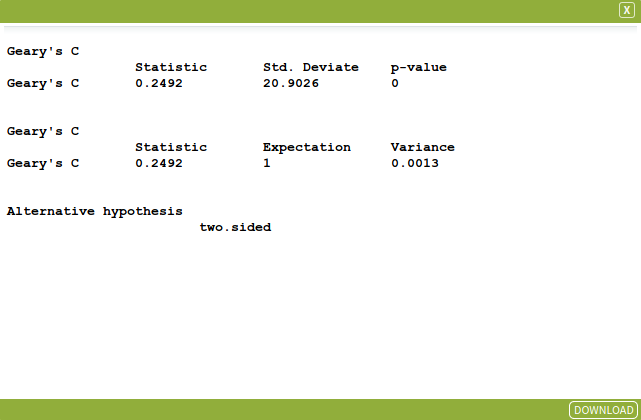Geary's C

Geary’s C is a measure of clustering (or spatial autocorrelation) of a variable, and is computed by (Cliff and Ord, 1981):

$C = (N-1){{\sum\nolimits_{i=1}^N \sum\nolimits_{j=1}^N w_{ij}(X_{i}-X_{j})^2}\over{2W\sum\nolimits_{i=1}^n(X_{i}-\overline{X})^2}}$

Where $X_i$ and $X_j$ are the values for regions $i$ and $j$ respectively, $\overline{X}$ is the mean of the variable, $W$ is the global sum of the weights, i.e. The spatial weight matrix that is employed by this component must be symmetric. Hence if the selected spatial weight matrix is not already symmetric, part of the process will include converting the spatial weight matrix so it becomes symmetric.

Given the null hypothesis is one of no global spatial autocorrelation, the expected value of Geary’s C equals 1. If C is larger than 1, the distribution of the variable being tested is characterised by negative spatial autocorrelation. If C is smaller than 1, then the distribution of the variable displays positive spatial autocorrelation. As with Moran’s I, the inference is based on z-values:

$Z_{c} = {{C - 1}\over{sd(C)}}$

While Moran’s I is based on the cross-products of the deviations from the mean, Geary’s C is based on the deviations in responses of each observation with one another. This in effect means that Moran’s I is more sensitive to extreme values and is more a global measure, whereas Geary’s C is more sensitive to differences between values in neighbouring areas.

SET UP

To demonstrate this tool in use, we will look at socio-economic disadvantage data in Greater Darwin to examine the extent of spatial-autocorrelation.

Select Greater Melbourne GCCSA as your area.

Select ABS – Socio-Economic Indexes for Areas (SEIFA) – The Index of Education and Occupation (SA2) 2016 as your dataset, selecting the following variables:

• IEO Score
• SA2 9-digit code 2016
• SA2 name 2016

Use the Spatialise Aggregated Dataset tool to Spatialise the dataset.Generate a Contiguous Spatial Weight Matrix for the spatialised dataset, using 1st order, row-standardised, Queen contiguity.

Inputs

Open the Geary’s C tool (Tools → Spatial Autocorrelation → Geary’s C) and enter the following parameters:

• Dataset Input: The dataset that contains the variable to be tested. Select the Spatialised Dataset that was generated in the above steps.
• Spatial Weights Matrix: The spatial weight matrix to be used. Select the generated Contiguous Spatial Weight Matrix that was generated in the above steps.
• Key Column: Specify the unique codes for your areas. Select SA2 9-digit code 2016.
• Variable: The variable to be tested. Select IEO Score.
• Alternative Hypothesis: Specifies the alternative hypothesis. Select two.sided.
• two.sided: a priori assumption that the difference between Ii and the expected E[Ii] is not equal to zero (spatial autocorrelation).
• greater: a priori assumption that Ii is greater than the expected E[Ii] (positive spatial autocorrelation).
• less: a priori assumption that Ii is less than the expected E[Ii] (negative spatial autocorrelation).
• Inference: Indicates the inference under which the variance of is calculated. Checked is randomisation, unchecked is normality. Tick for randomisation.

The input parameters are summarised in the image below, once complete click Run Tool.Outputs

Once the tool has run, click the Display button on the pop-up dialogue box that appears. This will open a window with the outputs of your Geary’s C calculation, which should look like the image below.

These outputs are:

• Geary’s C statistic value. This represents the spatial correlation of the data from 0. A value between 0 and 1 indicates positive spatial autocorrelation where values significantly larger than 1 indicate little spatial autocorrelation.
• The calculated standard deviate and p-value.
• Geary’s C’s expectation and variance.
• The alternative hypothesis and inference calculation set in the parameters.Centre of Full Employment and Equity. (2015). AURIN spatial statistics and econometrics e-tools help file (p. 10). University of Newcastle.
Cliff, A. D., & Ord, J. K. (1981). Spatial processes: Models & applications. Taylor & Francis.
Wikipedia. (2020). Geary’s C. https://en.wikipedia.org/wiki/Geary’s_C

Looking for Spatial Data?

You can browse the AURIN Data Discovery:How can you Create Impact?